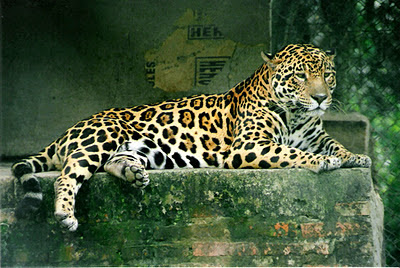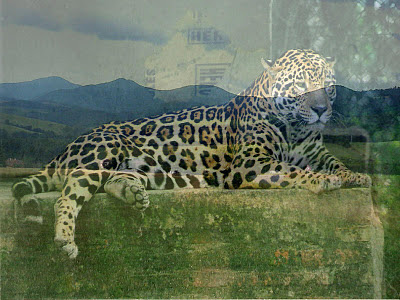## segunda-feira, 5 de dezembro de 2011

### Python: Image Processing with numpy, PIL (Python Image LIbrary) and PythonMagick

Below an example code with numpy and PIL for manipulate image.
Create it code and save like blend.py in directory.

`import numpy, Imageimport os"""Combine two image with a factor"""def addImages(img1, img2, factor):    #Convert to object type Image opened with Image.open(file) to array with numpy.    M, N = numpy.array(img1), numpy.array(img2)    #Type of value in M and N is uint8 that the type returned    #by Image.open() when open and create the object image.    A = M.astype('float')    B = N.astype('float')    #Combine the two images with the factor defined for user.    I = (A+B)/factor    #Return image. Before convert I in 'uint8' again and change    #for from array to image.    return Image.fromarray(I.astype('uint8'))`
`Examples for use of this module blend.py:`

`import blend `
`img1 =  Image.open(file_of_image)`
`img2 = Image.open(file_of_image)`
`#Resize image to ok combine.`
`I1 = img1.resize(x,y)`
`I2 = img2.resize(x,y)`
`#Combine with function in blend `
`I = blend.addImages(I1, I2, 2)`
`#Show image`
`I.show()`
`#Save your image created.`
`I.save(name_for_file_save)`

`Result bellow(the images that i use was get in wikimedia in wikipedia.org)`img1img2I - combine img1 and img2 with factor=2

`For use PythonMagick,`

`import PythonMagick#Open file of imageI = PythonMagick.Image("/home/wandeson/Imagens/pathera_onca.jpg")#View imageI.display()#Apply filter of oil paintI.oilPaint(0.8)`

`The result for oil paint effect in image is bellow`

`Links for other examples, docs, sources: `
`http://www.imagemagick.org/ `
www.pythonware.com/products/pil/
http://numpy.scipy.org